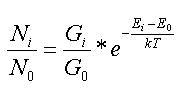## Occupancies calculator window

At this window the occupancies of e.g. electronic states can be calculated by their energies. This utility is not related to Chemcraft visualization possibilities. The occupancies are calculated by the Boltzman equation:Here Ni and N0 are the occupancies of the i-th and the first (lowest) state, Gi and G0 are the corresponding degeneracy coefficients, Ei is the energy of the i-th state, k is the Boltzman constant and T is the absolute temperature.

BACK